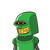# Use division algorithm to show that any positive odd integer is of the form 6q + 1,or 6q + 3 or 6q + 5, where q is som

Use division algorithm to show that any positive odd integer is of the form 6q + 1,

or 6q + 3 or 6q + 5, where q is some integer.​

### 1 thought on “Use division algorithm to show that any positive odd integer is of the form 6q + 1,<br /><br />or 6q + 3 or 6q + 5, where q is som”

1.Step-by-step explanation:

Let a be a given integer.

On dividing a by 6 , we get q as the quotient and r as the remainder such that

a = 6q + r, r = 0,1,2,3,4,5

when r=0

a = 6q,even no

when r=1

a = 6q + 1, odd no

when r=2

a = 6q + 2, even no

when r = 3

a=6q + 3,odd no

when r=4

a=6q + 4,even no

when r=5,

a= 6q + 5 , odd no

Any positive odd integer is of the form 6q+1,6q+3 or 6q+5.